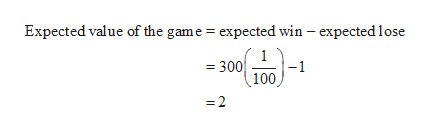# Use the fact that the mean of a geometric distribution is μ=1/p and the variance is σ2 =q/p2 A daily number lottery chooses two balls numbered 0 to 9. The probability of winning the lottery is 1/100. Let x be the number of times you play the lottery before winning the first time. (a) Find the mean, variance, and standard deviation. (b) How many times would you expect to have to play the lottery before winning? It costs \$1 to play and winners are paid \$300. Would you expect to make or lose money playing this lottery? Explain.

Question
144 views

Use the fact that the mean of a geometric distribution is μ=1/p and the variance is σ=q/p

A daily number lottery chooses two balls numbered 0 to 9. The probability of winning the lottery is 1/100. Let x be the number of times you play the lottery before winning the first time.

(a) Find the mean, variance, and standard deviation. (b) How many times would you expect to have to play the lottery before winning? It costs \$1 to play and winners are paid \$300. Would you expect to make or lose money playing this lottery? Explain.

check_circle

Step 1

It is given that x is the number of times to play the lottery before winning first time.

p = 1/100 and q = 99/100.

a).

The mean is calculated as follows.

Step 2

The variance and standard deviation calculated as follows.

Step 3

b). from part (a), the expected number of lotteries to play to get first win is 100.

Therefore, the expected ...help_outlineImage TranscriptioncloseExpected value of the game expected win - expected lose - 300 -1 100 =2 fullscreen

### Want to see the full answer?

See Solution

#### Want to see this answer and more?

Solutions are written by subject experts who are available 24/7. Questions are typically answered within 1 hour.*

See Solution
*Response times may vary by subject and question.
Tagged in

### Other